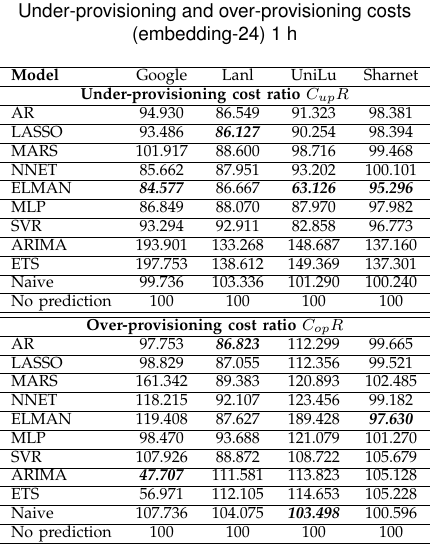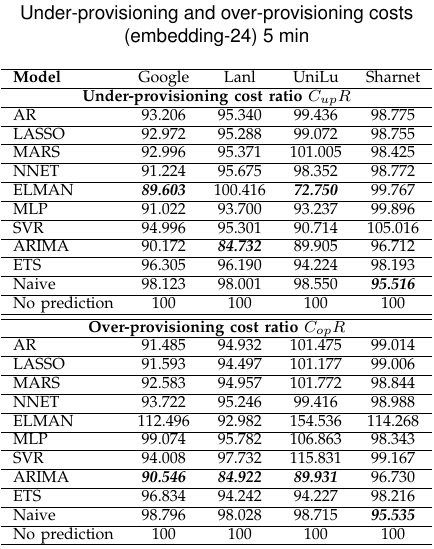# Measures of results:

An issue with measurement error as the mean square error of the root is not free of scale and can not be used to compare forecasts for different series. For series with strictly positive values is an effective alternative to normalize the value of the series, which produces percentage measures.

### RelMAE:

RelMAE is the {Mean Absolute Error} (MAE) normalized by the MAE of a reference method. We use the naïve forecast (where forecasts are equal to the last observed value) as benchmark MAEB. This measure provides additional information if the forecast performs better than a trivial method, so it performs a kind of sanity check for the forecasts, the methods with a RelMAE greater than one are not reasonable for forecasting (naïve forecasting is better). The RelMAE can be defined as: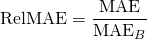### sMAPE:

Symmetric mean absolute percentage error (sMAPE) is an accuracy measure based on percentage or relative errors: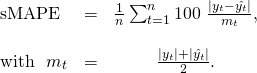We have chosen to display the results of this measure as a percentage.

### New cost function:

Errors overestimation and underestimation of the number of cores having different costs required in each case, so it is an asymmetrical problem. To take the measure both behavior we have implemented both cost functions where yt is the overall number of cores required at time t and zT is the overall number of cores supplied by the provider. We assume the period under evaluation is from time 1 up to m. The function that calculates the cost associated with the under-provisioning is: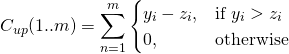The function that calculates the cost associated with the over-provisioning is: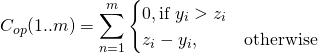We have chosen to present these results in a case relating to not applying any prediction algorithm. So we've divide the cost obtained in each case for each prediction algorithm for the cost associated with not applying any prediction algorithm.

# Tests results:

### Ljung-Box test

#### Non-seasonal study

In this case we can see how the null hypothesis is rejected in all cases except in ARIMA-5 UniLu embedding Google embedding 1h and ETS embedding-24 1h. In all cases where the p-value is less than 0.05 the null hypothesis is rejected and therefore there is some correlation between the different values of the residuals obtained from the corresponding models. This indicates that for all cases except the two mentioned above, it is recommended to continue studying other possible models.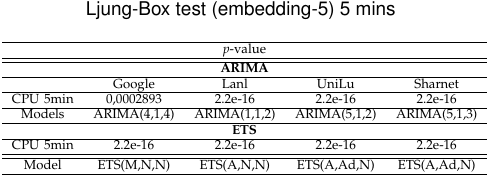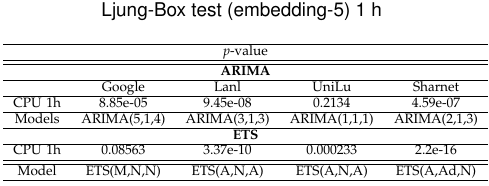#### Seasonal study

In this case we have only one hour predictions because we are working on the hypothesis of a daily seasonality. We can see only the case for Unilu not reject the null hypothesis, so we have chosen to continue to explore other models for all cases in order to achieve better results and to make a thorough study of the proposed methodology.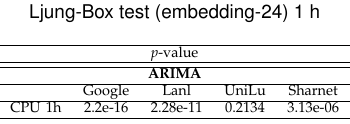### Teräsvirta test

We applied the test Teräsvirta on all processed input dataset. In all cases a p-value less than 0.05 is obtained. We have chosen to work with a confidence interval of 95%, so these p-value allow us to reject the null hypothesis of linear behavior of the data and using nonlinear prediction models are recommended.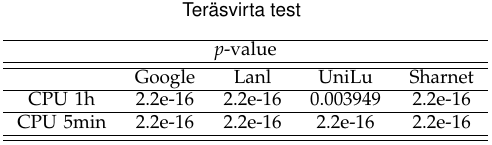# Results for RelMAE and sMAPE measures:

In this section we present the results to the predictions made about test sets for RelMAE and SMAPE measures, both for the case of non-seasonal and seasonal study.

### Non-seasonal study

We can see that there is no method to obtain better results in all cases, even for the same dataset and distinct prediction intervals. This behavior is expected for the different behaviors observed in each dataset.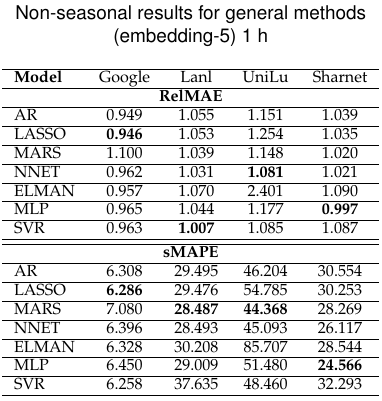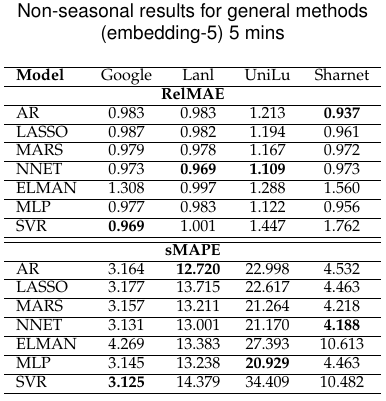### Seasonal study

In this case, it can be seen as non-seasonal studies provide better results than seasonal in most cases.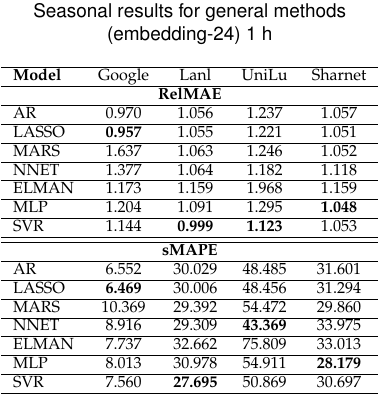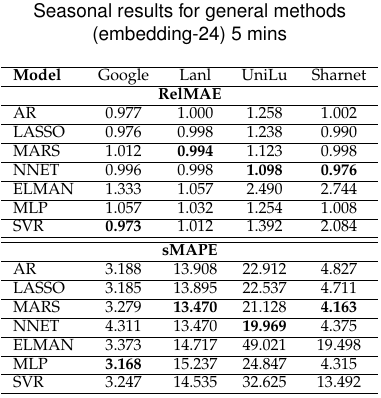# Results for new cost function:

In this section we present the results for the cost functions of over-provisioning and under-provisioning of cores, both for the case of non-seasonal and seasonal study. In most cases you can see a significant reduction in costs. The results of under-provisioning of cores are more relevant than those of over-provisioning of cores by compensation policy done by the companies.

### Non-seasonal study

In this case predictions for 1 hour intervals provide better results. This is largely explained by the attenuation of the sharp peaks in need of cores present in the time series studied.

###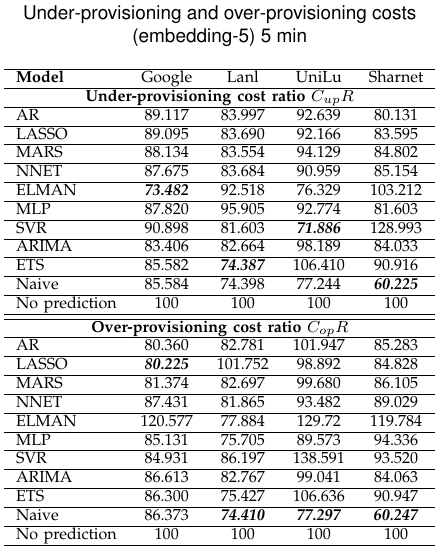### Seasonal study

As in the previous case it can be seen that results ls for non-seasonal study are better than those obtained for the case seasonal.

###